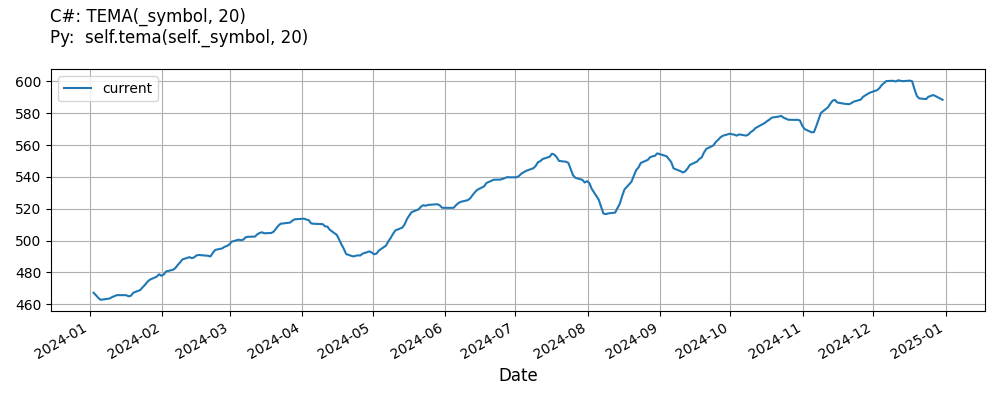# Supported Indicators

## Triple Exponential Moving Average

### Introduction

This indicator computes the Triple Exponential Moving Average (TEMA). The Triple Exponential Moving Average is calculated with the following formula: EMA1 = EMA(t,period) EMA2 = EMA(EMA(t,period),period) EMA3 = EMA(EMA(EMA(t,period),period),period) TEMA = 3 * EMA1 - 3 * EMA2 + EMA3

To view the implementation of this indicator, see the LEAN GitHub repository.

### Using TEMA Indicator

To create an automatic indicators for TripleExponentialMovingAverage, call the TEMA helper method from the QCAlgorithm class. The TEMA method creates a TripleExponentialMovingAverage object, hooks it up for automatic updates, and returns it so you can used it in your algorithm. In most cases, you should call the helper method in the Initialize method.

public class TripleExponentialMovingAverageAlgorithm : QCAlgorithm
{
private Symbol _symbol;
private TripleExponentialMovingAverage _tema;

public override void Initialize()
{
_tema = TEMA(_symbol, 20);
}

public override void OnData(Slice data)
{
{
// The current value of _tema is represented by itself (_tema)
// or _tema.Current.Value
Plot("TripleExponentialMovingAverage", "tema", _tema);

}
}
}
class TripleExponentialMovingAverageAlgorithm(QCAlgorithm):
def Initialize(self) -> None:
self.tema = self.TEMA(self.symbol, 20)

def OnData(self, slice: Slice) -> None:
# The current value of self.tema is represented by self.tema.Current.Value
self.Plot("TripleExponentialMovingAverage", "tema", self.tema.Current.Value)



The following reference table describes the TEMA method:

### TEMA()1/1

            TripleExponentialMovingAverage QuantConnect.Algorithm.QCAlgorithm.TEMA (
Symbol                           symbol,
Int32                            period,
*Nullable<Resolution>      resolution,
*Func<IBaseData, Decimal>  selector
)


Creates a new TripleExponentialMovingAverage indicator.

If you don't provide a resolution, it defaults to the security resolution. If you provide a resolution, it must be greater than or equal to the resolution of the security. For instance, if you subscribe to hourly data for a security, you should update its indicator with data that spans 1 hour or longer.

You can manually create a TripleExponentialMovingAverage indicator, so it doesn't automatically update. Manual indicators let you update their values with any data you choose.

Updating your indicator manually enables you to control when the indicator is updated and what data you use to update it. To manually update the indicator, call the Update method with time/number pair, or an IndicatorDataPoint. The indicator will only be ready after you prime it with enough data.

public class TripleExponentialMovingAverageAlgorithm : QCAlgorithm
{
private Symbol _symbol;
private TripleExponentialMovingAverage _tema;

public override void Initialize()
{
_tema = new TripleExponentialMovingAverage(20);
}

public override void OnData(Slice data)
{
if (data.Bars.TryGeValue(_symbol, out var bar))
{
_tema.Update(bar.EndTime, bar.Close);
}

{
// The current value of _tema is represented by itself (_tema)
// or _tema.Current.Value
Plot("TripleExponentialMovingAverage", "tema", _tema);

}
}
}
class TripleExponentialMovingAverageAlgorithm(QCAlgorithm):
def Initialize(self) -> None:
self.tema = TripleExponentialMovingAverage(20)

def OnData(self, slice: Slice) -> None:
bar = slice.Bars.get(self.symbol)
if bar:
self.tema.Update(bar.EndTime, bar.Close)

# The current value of self.tema is represented by self.tema.Current.Value
self.Plot("TripleExponentialMovingAverage", "tema", self.tema.Current.Value)



To register a manual indicator for automatic updates with the security data, call the RegisterIndicator method.

public class TripleExponentialMovingAverageAlgorithm : QCAlgorithm
{
private Symbol _symbol;
private TripleExponentialMovingAverage _tema;

public override void Initialize()
{
_tema = new TripleExponentialMovingAverage(20);
RegisterIndicator(_symbol, _tema, Resolution.Daily);
}

public override void OnData(Slice data)
{
{
// The current value of _tema is represented by itself (_tema)
// or _tema.Current.Value
Plot("TripleExponentialMovingAverage", "tema", _tema);

}
}
}
class TripleExponentialMovingAverageAlgorithm(QCAlgorithm):
def Initialize(self) -> None:
self.tema = TripleExponentialMovingAverage(20)
self.RegisterIndicator(self.symbol, self.tema, Resolution.Daily)

def OnData(self, slice: Slice) -> None:
# The current value of self.tema is represented by self.tema.Current.Value
self.Plot("TripleExponentialMovingAverage", "tema", self.tema.Current.Value)



The following reference table describes the TripleExponentialMovingAverage constructor:

### TripleExponentialMovingAverage()1/2

            TripleExponentialMovingAverage QuantConnect.Indicators.TripleExponentialMovingAverage (
string  name,
int     period
)


Initializes a new instance of the TripleExponentialMovingAverage class using the specified name and period.

### TripleExponentialMovingAverage()2/2

            TripleExponentialMovingAverage QuantConnect.Indicators.TripleExponentialMovingAverage (
int  period
)


Initializes a new instance of the TripleExponentialMovingAverage class using the specified period.

### Visualization

The following image shows plot values of selected properties of TripleExponentialMovingAverage using the plotly library.You can also see our Videos. You can also get in touch with us via Discord.